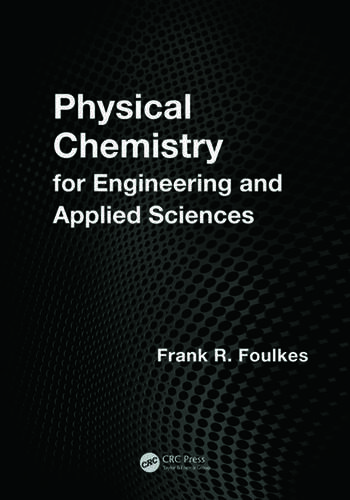Physical Chemistry for Engineering and Applied Sciences

1st Edition

Frank R. Foulkes

CRC Press
Published September 12, 2012
Textbook - 704 Pages - 159 B/W Illustrations
ISBN 9781466518469 - CAT# K15157

For Instructors Request Inspection Copy

was \$115.00

USD\$92.00

SAVE ~\$23.00

FREE Standard Shipping!

Preview

Summary

Physical Chemistry for Engineering and Applied Sciences is the product of over 30 years of teaching first-year Physical Chemistry as part of the Faculty of Applied Science and Engineering at the University of Toronto. Designed to be as rigorous as compatible with a first-year student’s ability to understand, the text presents detailed step-by-step derivations of the equations that permit the student to follow the underlying logic and, of equal importance, to appreciate any simplifying assumptions made or mathematical tricks employed.

In addition to the 600 exercises and end-of-chapter problems, the text is rich in worked non-trivial examples, many of which are designed to be inspiring and thought-provoking. Step-by-step derivation of all equations enables the student to smoothly follow the derivation by sight, and can be understood relatively easily by students with moderate skills and backgrounds in mathematics.

Clear and accessible, Physical Chemistry for Engineering and Applied Sciences includes:

• The answers to all of the 112 worked examples, 99 exercises following many of the worked examples, and 496 end-of-chapter problems
• Topics not normally seen in introductory physical chemistry textbooks (ionic reaction rates, activities and activity coefficients) or not regularly explained in much detail (electrochemistry, chemical kinetics), with an eye on industrial applications
• Special appendices that provide detailed explanations of basic integration and natural logarithms for students lacking a background in integral calculus
• An in-depth chapter on electrochemistry, in which activities and activity coefficients are used extensively, as required for accurate calculations

Instructors

We provide complimentary e-inspection copies of primary textbooks to instructors considering our books for course adoption.